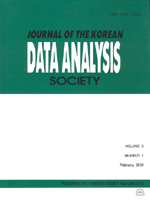상세검색
최근 검색어 전체 삭제
다국어입력
즐겨찾기0KCI등재 학술저널

# Meta Analysis on Effects of Bibliotherapy

• 등재여부 : KCI등재
• 2011.06
• 1091 - 1100 (10 pages)
• 5

The purpose of this study is to analyze effects of bibliotherapy using meta analysis. A total of 71 effect sizes have been calculated through the analysis of fifty one MA and Ph.D degree papers and journal articles published from 2006 to 2010; and the result of this study is as follows: First, mean effect size as a whole is as large as 1.326. Second, the difference in mean effect size between primary school students and middle (and high) school students is not statistically significant. Third, effect size of journal papers (1.172) turned out a little bigger than that of degree papers (1.135), which is not statistically significant. Fourth, according to the result of simple regression analysis carried on to determine whether or not program application term has a significant effect on effect size, its effect was found to be statistically insignificant, but as regression coefficient turned out negative even if it was tiny, it follows that there is need to adjust program application term properly. It is a limitation of this study that this article did not include unpublished results (degree papers and journal papers), but this study has a meaning in that it attempted to generate integrated results of bibliotherapy effects using meta analysis method.

1. Introduction

2. Concepts of Meta Analysis and Effect Size

3. Precedent Research Search and Data Evaluation

4. Analysis Result and Discussion

5. Conclusion

References